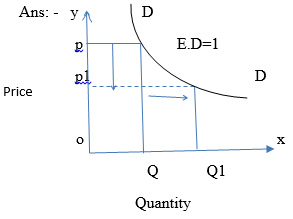Courses

# High Order Thinking Skills - Consumer's Equilibrium and Demand (Theory of Consumer Behaviour) Commerce Notes | EduRev

## Commerce : High Order Thinking Skills - Consumer's Equilibrium and Demand (Theory of Consumer Behaviour) Commerce Notes | EduRev

The document High Order Thinking Skills - Consumer's Equilibrium and Demand (Theory of Consumer Behaviour) Commerce Notes | EduRev is a part of the Commerce Course Economics Class 12.
All you need of Commerce at this link: Commerce

HOTS QUESTIONS

1. Give the formula for calculating the slope of the budget line.

Ans :-
It is equal to the ratio of the prices of the two commodities , i.e.,  Px / Py

2. Suppose a consumer’s preferences are monotonic. What can you say about his preference ranking over the bundles (10,10),(10,9) and (9,9)?

Ans :-
Consumer will monotonically prefer bundle (10,10) to (10,9) and (9,9) and also prefer bundle (10,9) to (9,9)

3. A rise in the income of the consumer leads to a fall in the demand for commodity ‘x’. What type of good is commodity ‘x’?

Ans :-
Inferior good

4. What do you mean by substitute and complementary goods? Give two examples each.

Ans :-
Substitute goods are those goods which can be used in place of each other. Ex. Tea and Coffee. Complementary goods are those goods which are used together to satisfy a given want. Ex : Car and petrol.

5. Mention one factor that causes a leftward shift of the demand curve.

Ans :-
Fall in income of  a consumer.

6. What causes a movement along the demand curve of a commodity?

Ans :-
When the price of a commodity changes and other factors remain constant, there will be movement along the demand curve.

7. What is demand function?

Ans: -
A demand function shows the functional relationship between the quantity demanded and the factors on which demand depends on.

8. Draw a demand curve with unitary elasticity.9. Define price elasticity of demand.
Ans :-
It refers to the degree of responsiveness of quantity demanded to change in price.

HOTS

1. Is the demand for the following elastic, moderate elastic, highly elastic? Give reasons.

1. Demand for petrol
2. Demand for text books
3. Demand for cars
4. Demand for milk

Ans :- i) Demand for petrol is moderately elastic , because when the price of the petrol goes up , the consumer will reduce the use of it.

ii)Demand for text books is completely inelastic. In case of text books, even a substantial change in price leaves the demand unaffected.

iii) Demand for cars is elastic. It is a luxury good, when the price of the car rises, the demand for the car comes down.

iv) Demand for milk is elastic, because price of the milk increases then the consumer purchase less quantity milk.

2. What is the relationship between slope and elasticity of a demand curve?

Ans :- The formula of Ed = ΔQ / ΔP * P / Q

The formula for the slope of the demand curve is, slope = ΔP / ΔQ

The relationship between slope and elasticity of demand is Ed= 1/slope * P/Q

Offer running on EduRev: Apply code STAYHOME200 to get INR 200 off on our premium plan EduRev Infinity!

## Economics Class 12

224 videos|237 docs|54 tests

,

,

,

,

,

,

,

,

,

,

,

,

,

,

,

,

,

,

,

,

,

;